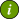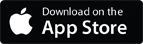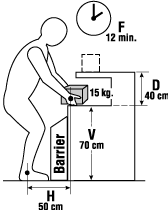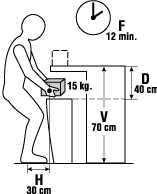### OSH Answers Fact SheetsEasy-to-read, question-and-answer fact sheets covering a wide range of workplace health and safety topics, from hazards to diseases to ergonomics to workplace promotion. MORE ABOUT >Search all fact sheets:

Type a word, a phrase, or ask a question

# Horizontal Distance Multiplier Factor

## How can the horizontal distance affect the weight limit?

Example: A worker lifts 15 kg boxes from the table to the shelf five times an hour. Notice that there is a barrier between the worker and the box.To calculate the recommended weight limit (RWL) for the task:

• Determine the weight of the load.

Weight equals 15 kg

H (Horizontal Distance) - 50 cm
V (Vertical Distance) - 70 cm
D (Lifting/Carrying Distance) - 40 cm
F (Frequency) - 12 min
A (Angle) - 0°
C (Coupling/quality of grip) - fair

The revised NIOSH Lifting Equation is: 23 Kg x HM x VM x DM x AM x FM x CM= RWL

23 Kg x 0.50 x 0.99 x 0.93 x 1.00 x 1.00 x 1.00 = 10.59 kg

• Compare the weight of the load against determined Recommended Weight Limit for the task:

The recommended weight is 10.59 kg, which is less than the current weight at 15 kg.

### Conclusion

The weight of the load is 15 kg, which is higher than the calculated recommended weight limit of 10.59 kg.

Therefore, this task is likely to increase the risk of a lifting related injury.

### Recommendations

The critical component is H, the horizontal distance of the box from worker's body.

• Remove the barrier to allow worker to get closer to the box (horizontal distance is now 30 cm which uses a factor of 0.83).The revised NIOSH Lifting Equation is: 23 kg x HM x VM x DM x AM x FM x CM = RWL

23 kg x 0.83 x 0.99 x 0.93 x 1.00 x 1.00 x 1.00 = 17.58 kg

• Compare weight of the load against Weight Limit in new layout.

The weight of the load at 15 kg is now lower than the recommended weight limit of 17.58 kg.

Therefore, MOST WORKERS CAN SAFELY PERFORM THE TASK.

Document last updated on May 27, 2021